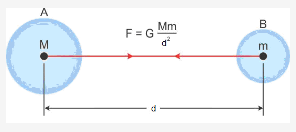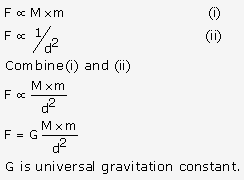# Derive a mathematical expression for the Newton's law of gravitation

Derive a mathematical expression for the Newton’s law of gravitation.

Every object in this universe attracts every other object with a force which is directly proportional to the product of their masses and inversely proportional to the square of the distance between their centres. The direction of force is along the line joining the centres of two objects.Consider two bodies A and B of masses M and m respectively and ‘d’ is the distance between then centres. Let F be the force by which they mutually attract each other